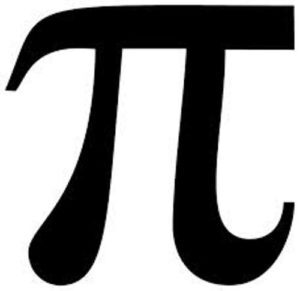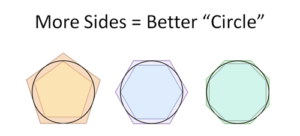## The Joy of π

by | Mar 14, 2018Happy March 14th: Pi Day!

Pi, of course, is the ratio of a circle’s circumference to it’s diameter. Pi is an irrational number, meaning it cannot be written as a simple fraction. Instead, it is expressed with an infinite, non-repeating decimal. Below is a discussion of how Pi is calculated followed by a few fun facts about Pi.

HOW IS PI CALCULATED? Pi has long fascinated me – specifically, how is Pi calculated? It is simple enough to measure the circumference of a circle as well as it’s diameter and find that Pi is equal to about 3 and a bit.  But, how did we figure out all the rest of the decimals with precision?

The earliest records of Pi date from 3,000 – 4,000 years ago came from trial and error measurements without doing any math by the Babylonians and Egyptians. These approximations were 3.125 and 3.1605. Pretty close.

Around 250 B.C.E. mathematician Archimedes drew polygons around the interior and exterior circumference of a circle and used the areas created by those polygons to estimate the upper and lower bounds of Pi. He was able to get Pi down to an estimate of 3.14.  Over the next millennia, mathematicians used polygons with more and more sides to produce more digits of Pi. In 1630 C.E. 38 digits were discovered using the polygon technique. Each additional digit took years and years of calculations.A breakthrough in calculating Pi occurred with the mathematical technique of an “infinite series” and the discovery of calculus. (As an interesting aside, check out this IFOD: Was Mathematics Invented or Discovered?) Newton used the infinite series technique calculate to Pi to 15 decimals. From the mid 1600s until the 1900s a number of different calculus formulas were “discovered” to take Pi out to a greater number of decimals. The formulas used are iterative algorithms which use the result of a formula as the input to run the formula again, and again, and so on. As an example, here’s the formula mathematician John Machin used to calculate 100 digits of Pi in 1706:The most number of digits calculated without a computer was 620 digits in 1956. Currently, about 12 Trillion digits have been discovered using very fast computers (but it takes months and lots of disk space) — UPDATE — as of March 2019, about 31 Trillion digits have been discovered.

How many digits of Pi do we need? NASA uses 3.141592653589793 for Pi in making its calculations because 15 digits of Pi is ridiculously precise. The Earth has a diameter of 7,926 miles. Using Pi of 15 decimal places will give you the correct circumference of Earth within the width of a single molecule!

What does the Bible say about Pi? 1 Kings 7:23: “Also he made a molten sea of ten cubits from brim to brim, round in compass, and five cubits the height thereof; and a line of thirty cubits did compass it round about.” 2 Chronicles 4:2 also provides the same measurements. This states that the ratio is 3, which has sparked a lot of theological debates given that 3 is not that close of an approximation.

Memorizing Pi: In 2006 Akira Haraguchi recited over 100,000 digits of pi from memory at a public event near Tokyo but was not verified by Guinness Book. It took him 16hrs 30mins. Haraguchi says that he’s recited 111,700 digits on his own. The record verified by the Guinness Book of World Records is Rajveer Meena who recited nearly 70,000 digits while blindfolded. It took him 10 hours.

Finally – a shout out to those of you who live in the St. Louis region and the 314 Area Code!!!! If you know someone in the 314 area code, maybe give them a call on Pi Day? Here’s a pretty interesting IFOD on how area codes are assigned: Area Codes and Elitism

This site uses Akismet to reduce spam. Learn how your comment data is processed.## Archives## Subscribe To The IFOD

Get the Interesting Fact of the Day delivered twice a week. Plus, sign up today and get Chapter 2 of John's book The Uncertainty Solution to not only Think Better, but Live Better. Don't miss a single post!